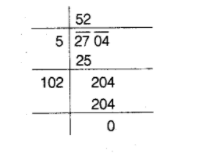# A hall has a capacity of 2704 seats.

Question:

A hall has a capacity of 2704 seats. If the number of rows is equal to the number of seats in each row, then find the number of seats in each row.

Solution:

Let the number of seats in each row be $x$.

Then, number of rows $=$ Number of seats in each row $=x$

$\therefore$ Total seats $=x \times x=x^{2}$

According to the question,$x^{2}=2704$

$\Rightarrow \quad x=\sqrt{2704}=\sqrt{52 \times 52}$

$\Rightarrow \quad x=52$

Hence, there are 52 seats in each row.